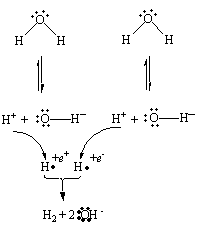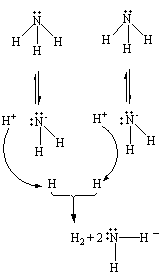Active Metal Reactions

Active Metal Reactions with Oxygen

The method used to predict the products of reactions of the main group metals is simple, yet remarkably powerful. Exceptions to its predictions arise, however, when very active metals react with oxygen, which is one of the most reactive nonmetals.

Lithium is well behaved. It reacts with O2 to form an oxide that contains the O2- ion.

4 Li(s) + O2(g)2 Li2O(s)

Sodium, however, reacts with O2 under normal conditions to form a compound that contains twice as much oxygen.

2 Na(s) + O2(g)Na2O2(s)

Compounds such as Na2O2 that are unusually rich in oxygen are called peroxides. The prefix per- means "above normal" or "excessive." Na2O2 is a peroxide because it contains more than the usual amount of oxygen. Peroxides contain the O22- ion.

The formation of sodium peroxide can be explained by assuming that sodium is so reactive that the metal is consumed before each O2 molecule can combine with enough sodium to form Na2O. This explanation is supported by the fact that sodium reacts with O2 in the presence of a large excess of the metalor a limited amount of O2to form the oxide expected when this reaction goes to completion.

4 Na(s) + O2(g)2 Na2O(s)

It is also consistent with the fact that the very active alkali metalspotassium, rubidium, and cesiumreact so rapidly with oxygen they form superoxides, in which the alkali metal reacts with O2 in a 1:1 mole ratio.

K(s) + O2(g)KO2(s)

Potassium superoxide forms on the surface of potassium metal, even when the metal is stored under an inert solvent. As a result, old pieces of potassium metal are potentially dangerous. When someone tries to cut the metal, the pressure of the knife pushing down on the area where the superoxide touches the metal can induce the following reaction.

KO2(s) + K(s)K2O(s)

Because potassium oxide is more stable than potassium superoxide, this reaction gives off enough energy to boil potassium metal off the surface, which reacts explosively with the oxygen and water vapor in the atmosphere.Active Metals and Water

The reactivity of the active metals can be demonstrated by dropping pieces of lithium, sodium, and potassium into water. Lithium reacts slowly with water, sodium reacts much more rapidly, and potassium reacts violently.

The model used to predict the products of reactions between main group metals and nonmetals can be extended to predict what will happen when these metals react with covalent compounds.

Example: Let's start by applying this model to the reaction between sodium metal and water.

Na(s) + H2O(l)?

In the course of this reaction, each sodium atom will lose an electron to form an Na+ ion.

NaNa+ + e-

The figure below shows what happens when these electrons are picked up by neighboring water molecules.Hydrogen atoms in a +1 oxidation state pick up electrons to form neutral hydrogen atoms, which combine to form H2 molecules.

2 H+ + 2 e-H2

Subtracting a positively charged H+ ion from a neutral H2O molecule leaves an OH- ion. The reaction that occurs when water molecules gain electrons can therefore be written as follows.

2 H2O + 2 e-H2 + 2 OH-

The reaction between sodium and water can be divided into two halves. One half-reaction describes what happens when sodium atoms lose electrons. The other half-reaction describes what happens when water molecules gain these electrons. By combining these half-reactions so that electrons are neither created nor destroyed, we can obtain an overall equation for the reaction.

 2 [NaNa+ + e-] 2 H2O + 2 e-H2 + 2 OH- ¯¯¯¯¯¯¯¯¯¯¯¯¯¯¯¯¯¯¯¯¯¯¯¯¯¯¯¯ 2 Na + 2 H2O2 Na+ + 2 OH- + H2

The balanced equation for this reaction can be written as follows.

2 Na(s) + 2 H2O(l)2 Na+(aq) + 2 OH-(aq) + H2(g)Active Metals and Ammonia

To predict what happens when an alkali metal, such as potassium, reacts with liquid ammonia (NH3) we can start by noting that the potassium atoms will lose one electron each.

KK+ + e-

The figure below shows what happens to these electrons.Hydrogen atoms in a +1 oxidation state gain electrons to form neutral hydrogen atoms, which combine to form H2 molecules.

2 H+ + 2 e-H2

Removing an H+ ion from an NH3 molecule leaves a negatively charged NH2- ion. The following equation therefore describes what happens when ammonia gains electrons.

2 NH3 + 2 e-H2 + 2 NH2-

Combining the two halves of this reaction so that electrons are conserved gives the overall equation for the reaction.

 2 [KK+ + e-] 2 NH3 + 2 e-H2 + 2 NH2- ¯¯¯¯¯¯¯¯¯¯¯¯¯¯¯¯¯¯¯¯¯¯¯¯¯¯¯ 2 K + 2 NH32 K+ + 2 NH2- + H2

The balanced equation for this reaction is written as follows.

2 K(s) + 2 NH3(l)2 K+(sol) + 2 NH2-(sol) + H2(g)

(The symbol sol in this equation indicates that the K+ and NH2- ions in this solution are solvated by neighboring NH3 molecules.)